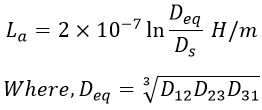# Inductance of 3-Phase Line with Unsymmetrical Spacing and Transposed

Inductance is one of the transmission line parameters. As the transmission lines carry alternating currents which produce alternating magnetic fluxes, the number of flux linkages with the conductor changes and hence causes inductance to be present in the line which is the ratio of total magnetic flux linkages to the current flowing through it. This inductance limits the power transfer capability of the line.

Hence, it is essential to calculate the inductance of the transmission line for analysis. let us see the expression for the inductance of the 3-phase transmission line when the conductors are unequally spaced.

## Inductance of Unsymmeterically Spaced 3-Phase Transmission Line :

Consider a 3-phase overhead transmission line with phase conductors a,b, and c of radius r each spaced unsymmetrically such that the distance between the three conductors is D1, D2, and D3 as shown in the figure below. Let Ia, Ib, and Ic be the currents flowing in the conductors a, b, and c respectively.

Assume that the whole system is balanced which leads to the flow of equal currents in the conductors i.e., Ia = Ib = Ic = I(say). The currents Ia, Ib, and Ic are displaced at 120° apart from each other. If Ia is taken as the reference phasor then the currents are given by,

We know that the flux linkage of any conductor, in a group of conductors, is due to its own current and currents in the other conductors. Therefore, flux linkage of the conductor 'a' is due to its own current and currents in the conductors 'b' and 'c', and it is given by,

#### Similarly, flux linkages of conductors 'b' and 'c' is given by,Where, r' = Geometric mean radius (GMR) of conductor = 0.07788 × r. Substituting the values of Ia, Ib, and Ic in the above equation we get,We know that the inductance La is given by,Similarly, the inductance due to the conductors 'b' and 'c' can be calculated and they are given by,When conductors are unsymmetrically spaced in a 3-phase line, the flux linkage and inductance per phase are not identical, and knowing the inductance of each phase becomes complicated and it results in an unbalanced circuit.

The equilibrium in the circuit can be retained by shifting the places of the conductor at every period of time such that the conductors take the initial place of every conductor at the same distances.

Such an arrangement of the conductor's position obtained by shifting their places is called transposition. By transposition, we can obtain almost the same inductance between the conductors. Let us see the expression for inductance when the 3-phase line is transposed.

## Inductance of Unsymmeterically Spaced 3-Phase Transmission Line When Transposed :

The simple configuration of a 3-phase conductor is shown in the figure. As the conductors are transposed, so their position in the transposition cycle would be as shown in the figure below.

When the conductors are connected in parallel, it results in low inductance keeping the distance between phases as small as possible. For deriving the inductance per phase of 3-phase conductors placed unsymmetrically, we need to determine the flux linkage of each conductor for every position it occupies in a transposition cycle. After that, we can determine the average flux linkages. So, the flux linkage of phase 'a' in position 1 is given as,

#### When 'a' is in position 2, 'b' in position 3 and 'c' in position 1,When 'a' is in position 3, 'b' in position 1, and 'c' in position 2,The average value of flux linkage of a single-phase 'a' is,We know that,Therefore, the average inductance per phase is,Where,Deq = Geometric mean of three distances of unsymmetrical lineDs = GMR (geometric mean radius) of the conductor.

The above expression is the inductance per phase of a 3-phase transmission line with unsymmetrical spacing but lines are transposed. Nowadays the transposition of conductors is made at switching stations to balance inductance.

Do not enter any spam links and messages